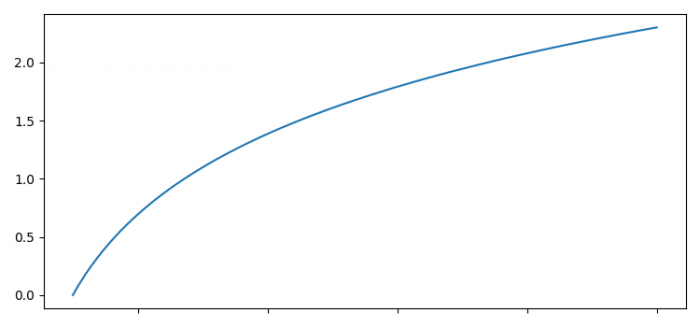# How to set the unit length of an axis in Matplotlib?

To set the unit length of an axis in Matplotlib, we can use xlim or ylim with scale factor of the axes, i.e., of unit length times.

## steps

• Set the figure size and adjust the padding between and around the subplots.

• Create x and y data points using numpy.

• Plot the x and y data points using plot() method.

• Get the x and y axes, limit range.

• Use xlim and ylim methods to set the unit length scale.

• To display the figure, use show() method.

## Example

import matplotlib.pyplot as plt
import numpy as np

plt.rcParams["figure.figsize"] = [7.50, 3.50]
plt.rcParams["figure.autolayout"] = True

x = np.linspace(1, 10, 100)
y = np.log(x)

plt.plot(x, y)
axis_scale = 2

xmin, xmax = plt.xlim()
ymin, ymax = plt.ylim()

plt.xlim(xmin * axis_scale, xmax * axis_scale)
plt.ylim(ymin * axis_scale, ymax * axis_scale)

plt.show()

## Output